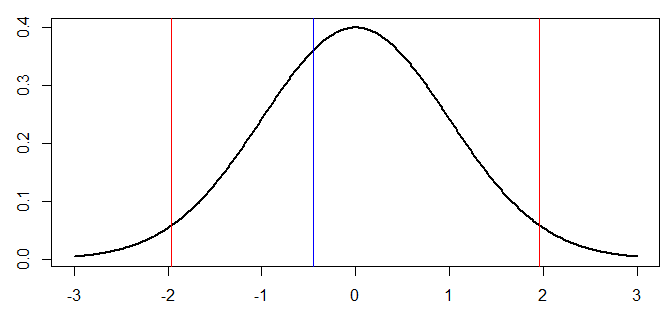Want to share your content on R-bloggers? click here if you have a blog, or here if you don't.

This morning, in our mathematical statistics course, we’ve been discussing the ‘proportion test‘, i.e. given a sample of Bernoulli trials, with , we want to test

against

A natural test (which can be related to the maximum likelihood ratio test) is  based on the statistic

The test function is here

To get the bounds of the acceptance region, we need the distribution of , under . Consider here a numerical application

```n=20
p=.5
set.seed(1)
echantillon=sample(0:1,size=n,
prob=c(1-p,p),
replace=TRUE)```
• the asymptotic distribution

The first (and standard idea) is to use the central limit theorem, since

So, under ,

Then  while . The acceptance region is then between the two red lines, below,

```T=sqrt(n)*(mean(echantillon)-.5)/
sqrt(mean(echantillon)*
(1-mean(echantillon)))
u=seq(-3,3,by=.01)
v=dnorm(u)
plot(u,v,type="l",lwd=2)
abline(v=qnorm(.025),col="red")
abline(v=qnorm(.975),col="red")
abline(v=T,col="blue")```• the exact distribution

Here we use the fact that

Using transformation of the ‘density’, we can (at least numerically) compute the (exact) distribution of

```u=seq(-3,3,by=.01)
v=sqrt(.5*(1-.5))*n*dbinom(round(
(sqrt(.5*(1-.5))*u/sqrt(n)+.5)*n),
size=n,prob=.5)/sqrt(n)```

Here I used a round value, it guess it would be better with a floor function, but here the graph looks symmetric (which is something I like)

```abline(v=sqrt(n)*(qbinom(.025,size=n,prob=.5)/n-.5)/sqrt(.5*(1-.5)),col="red")
abline(v=sqrt(n)*(qbinom(.975,size=n,prob=.5)/n-.5)/sqrt(.5*(1-.5)),col="red")
lines(u,v,type="s")```• distribution based on Monte Carlo simulations

Probably more interesting, here we do not use the fact that we might know the distribution of the mean. We just generate random samples, under , and then compute ,

```T=rep(NA,1000)
for(i in 1:1000){
x=sample(0:1,size=n,
prob=c(1-.5,.5),
replace=TRUE)
m=mean(x)
T[i]=(m-.5)/sqrt(m*(1-m))*sqrt(n)}
lines(density(T),lwd=2)
abline(v=quantile(T,.025),col="red")
abline(v=quantile(T,.975),col="red")```